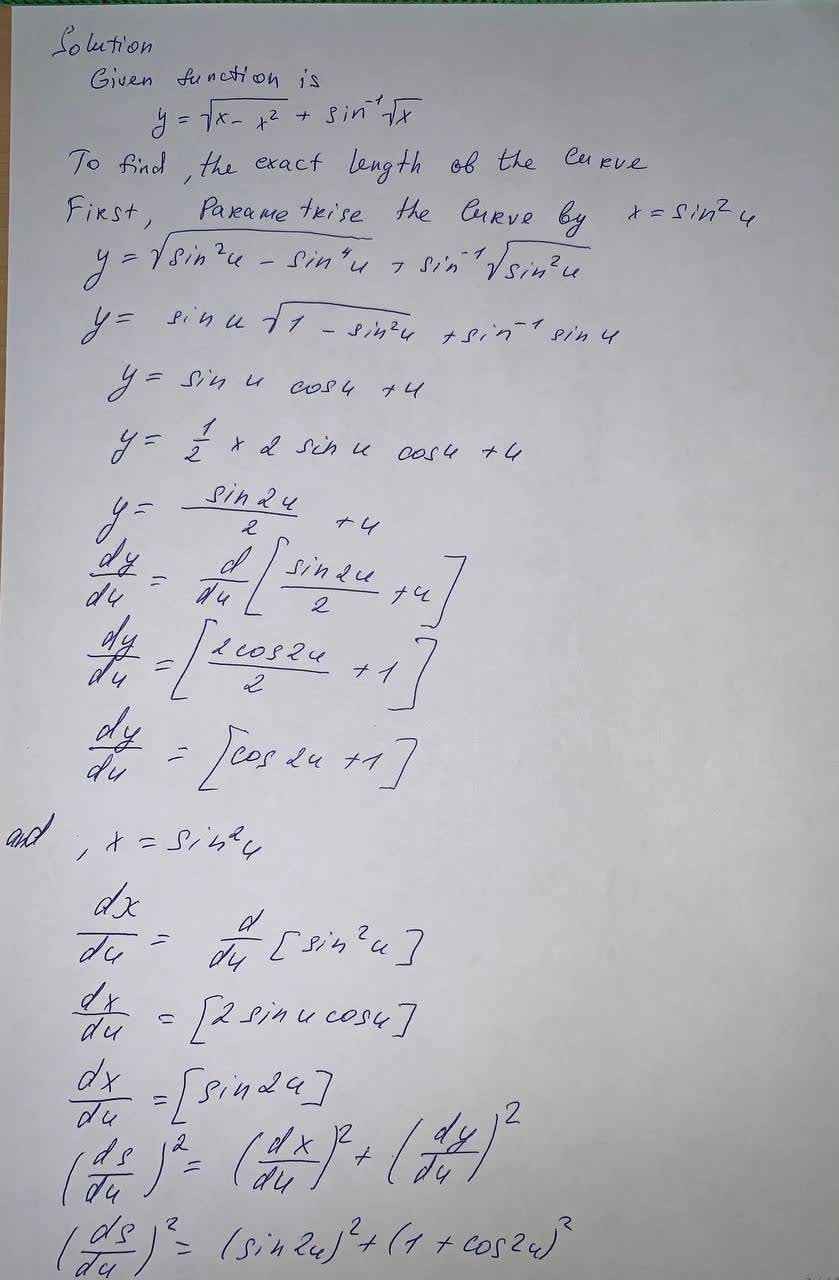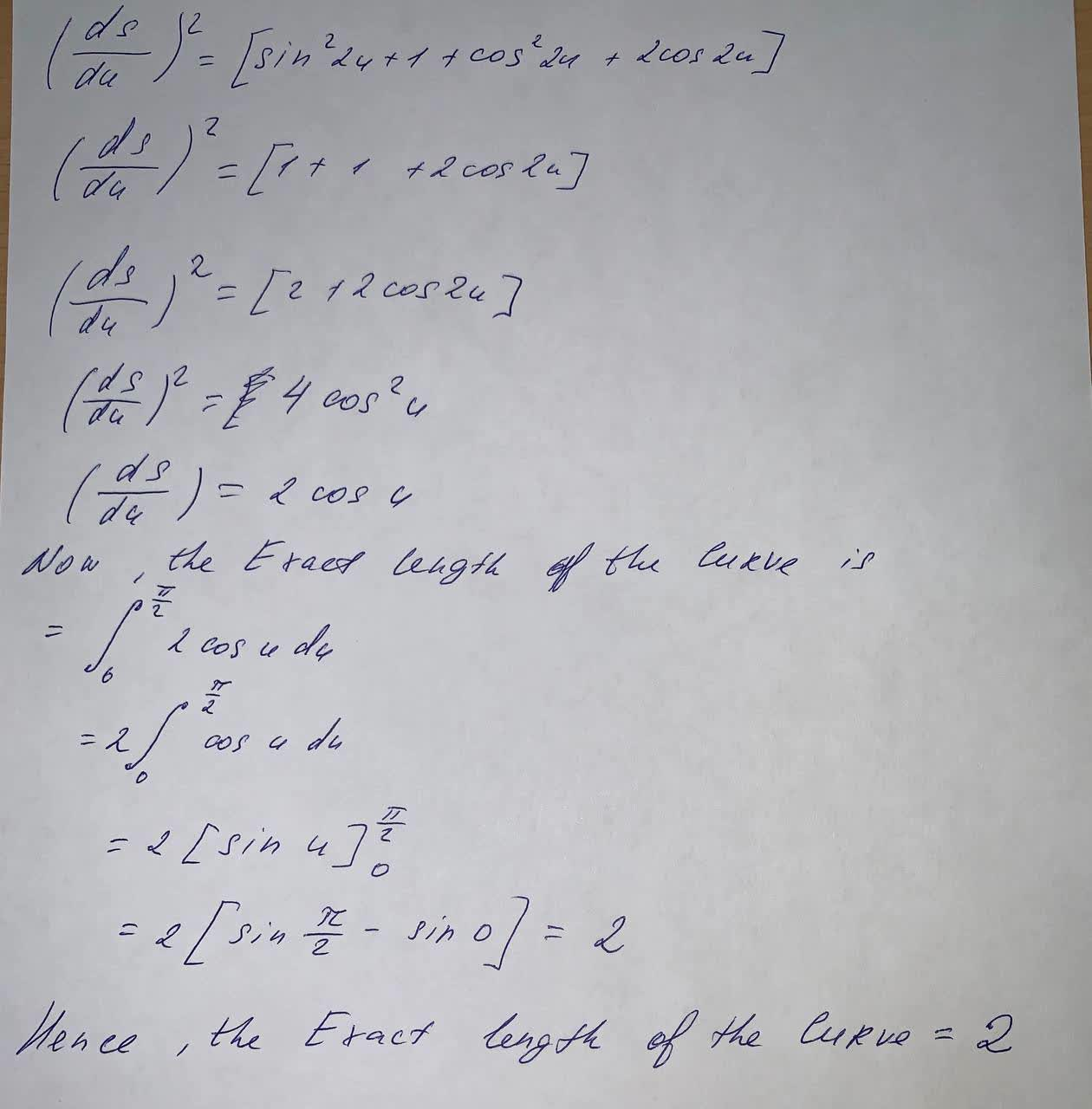#### Didn’t find what you are looking for?

Question# Find the exact length of the curve. y=\sqrt{x-x^2}+\sin ^{-1}\sqrt{x}

Integrals
ANSWEREDFind the exact length of the curve.
$$y=\sqrt{x-x^2}+\sin ^{-1}\sqrt{x}$$